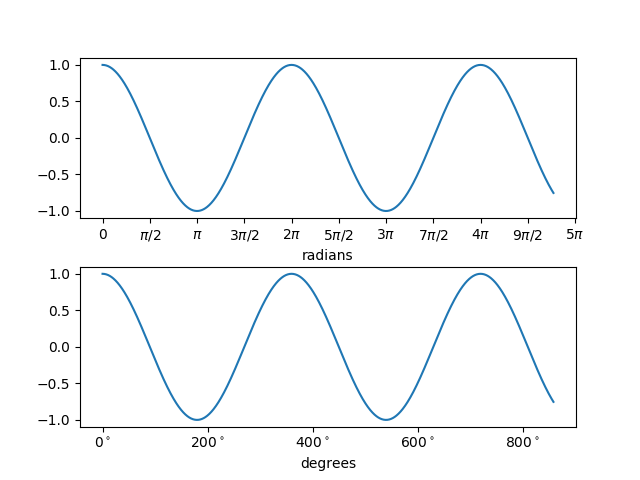Travis-CI:### Related Topics

Plot with radians from the basic_units mockup example package.

This example shows how the unit class can determine the tick locating, formatting and axis labeling.

This example requires `basic_units.py````import numpy as np
from basic_units import radians, degrees, cos
from matplotlib.pyplot import figure, show

x = [val*radians for val in np.arange(0, 15, 0.01)]

fig = figure()